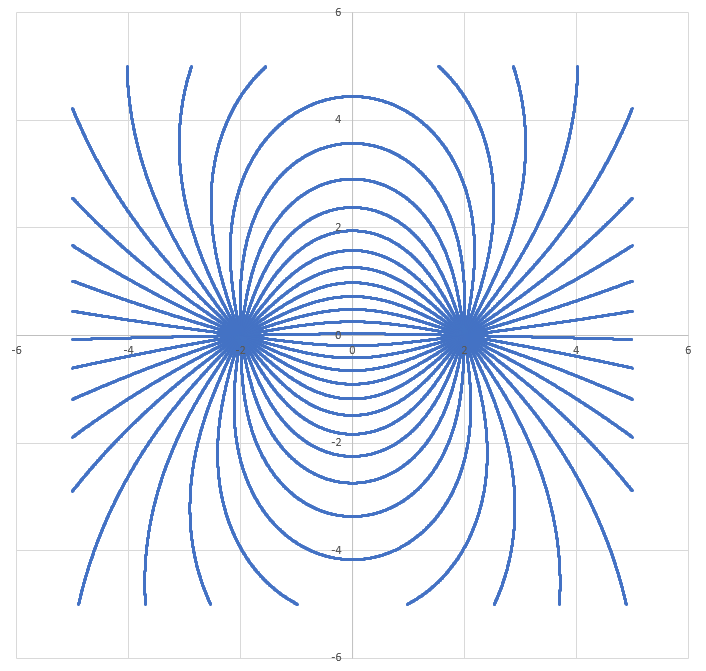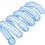# Plotting Electric Dipole Field Lines

The net electric field vector can be calculated at any point in the vicinity of an electric dipole. Each point in space can therefore be associated with a directional unit vector indicating the field's direction. We can follow these vectors, taking tiny steps along the field lines to get from one charge to the other, plotting the field lines as we go. The high-level process is:

1) Set up many departure points around the first charge, arrayed in a circle. Start points are initialized a small distance away from charge 1, at various angles. Each start point is associated with a distinct field line which starts near charge 1 and ends near charge 2.

2) To move from one point on the field line to another, first calculate the net electric field vector at the point. Then create a unit-direction vector based on the E-field. Then move a tiny distance "epsilon" in the direction of the unit vector to get to the next point on the field line. Continue along a particular field line until arriving at charge 2.

3) Upon arriving at charge 2, end the present field line, initialize a new departure point around charge 1, and trace out the next field line.

Example Plot:

Interestingly, we can see that the lines are closer where the field should be stronger. This feature "emerges" from the plotting process, without having to be explicitly coded.Code:

import math

# Constants

k = 3.7

q1 = 1.0
q2 = -1.0

x1 = -2.0
y1 = 0.0

x2 = 2.0
y2 = 0.0

delta = 10.0**(-3.0)

# Departure angle resolution

dtheta = 2.0 * math.pi / 40.0

theta = dtheta / 7.0

rst = 0.01

# Count decouples sim resolution from print resolution

count = 0

while theta <= 2.0 * math.pi:    # Departure points all around charge 1

x = x1 + rst * math.cos(theta)
y = y1 + rst * math.sin(theta)

d2 = 999999999.0

while d2 > rst:             # Follow field lines until arriving at charge 2

dx1 = x - x1
dy1 = y - y1

dx2 = x - x2
dy2 = y - y2

d1 = math.hypot(dx1,dy1)
d2 = math.hypot(dx2,dy2)

ux1 = dx1 / d1
uy1 = dy1 / d1

ux2 = dx2 / d2
uy2 = dy2 / d2

E1mag = k*q1 / (d1**2.0)
E2mag = k*q2 / (d2**2.0)

Ex1 = E1mag * ux1
Ey1 = E1mag * uy1

Ex2 = E2mag * ux2
Ey2 = E2mag * uy2

Ex = Ex1 + Ex2
Ey = Ey1 + Ey2

Emag = math.hypot(Ex,Ey)

uEx = Ex / Emag
uEy = Ey / Emag

x = x + delta * uEx
y = y + delta * uEy

if (math.fabs(x) < 5.0) and (math.fabs(y) < 5.0) and (count % 10 == 0):

print x,y

count = count + 1

theta = theta + dthetaNote by Steven Chase
3 years, 5 months ago

This discussion board is a place to discuss our Daily Challenges and the math and science related to those challenges. Explanations are more than just a solution — they should explain the steps and thinking strategies that you used to obtain the solution. Comments should further the discussion of math and science.

When posting on Brilliant:

• Use the emojis to react to an explanation, whether you're congratulating a job well done , or just really confused .
• Ask specific questions about the challenge or the steps in somebody's explanation. Well-posed questions can add a lot to the discussion, but posting "I don't understand!" doesn't help anyone.
• Try to contribute something new to the discussion, whether it is an extension, generalization or other idea related to the challenge.

MarkdownAppears as
*italics* or _italics_ italics
**bold** or __bold__ bold
- bulleted- list
• bulleted
• list
1. numbered2. list
1. numbered
2. list
Note: you must add a full line of space before and after lists for them to show up correctly
paragraph 1paragraph 2

paragraph 1

paragraph 2

[example link](https://brilliant.org)example link
> This is a quote
This is a quote
    # I indented these lines
# 4 spaces, and now they show
# up as a code block.

print "hello world"
# I indented these lines
# 4 spaces, and now they show
# up as a code block.

print "hello world"
MathAppears as
Remember to wrap math in $$ ... $$ or $ ... $ to ensure proper formatting.
2 \times 3 $2 \times 3$
2^{34} $2^{34}$
a_{i-1} $a_{i-1}$
\frac{2}{3} $\frac{2}{3}$
\sqrt{2} $\sqrt{2}$
\sum_{i=1}^3 $\sum_{i=1}^3$
\sin \theta $\sin \theta$
\boxed{123} $\boxed{123}$